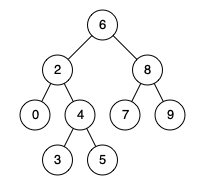### LeetCode• ㊗️
• 大家
• offer
• 多多！

## Problem

Given a binary search tree (BST), find the lowest common ancestor (LCA) of two given nodes in the BST.

According to the definition of LCA on Wikipedia: “The lowest common ancestor is defined between two nodes p and q as the lowest node in T that has both p and q as descendants (where we allow a node to be a descendant of itself).”

Given binary search tree: root = [6,2,8,0,4,7,9,null,null,3,5]Example 1:

Input: root = [6,2,8,0,4,7,9,null,null,3,5], p = 2, q = 8
Output: 6
Explanation: The LCA of nodes 2 and 8 is 6.


Example 2:

Input: root = [6,2,8,0,4,7,9,null,null,3,5], p = 2, q = 4
Output: 2
Explanation: The LCA of nodes 2 and 4 is 2, since a node can be a descendant of itself according to the LCA definition.


Constraints:

All of the nodes' values will be unique.
p and q are different and both values will exist in the BST.


## Code

/**
* Definition for a binary tree node.
* public class TreeNode {
*     int val;
*     TreeNode left;
*     TreeNode right;
*     TreeNode(int x) { val = x; }
* }
*/
class Solution {
public TreeNode lowestCommonAncestor(TreeNode root, TreeNode p, TreeNode q) {
if(root.val > p.val && root.val > q.val){
return lowestCommonAncestor(root.left, p, q);
} else if(root.val < p.val && root.val < q.val){
return lowestCommonAncestor(root.right, p, q);
} else {
return root;
}
}
}

# Definition for a binary tree node.
# class TreeNode:
#     def __init__(self, x):
#         self.val = x
#         self.left = None
#         self.right = None

class Solution:
def lowestCommonAncestor(self, root: 'TreeNode', p: 'TreeNode', q: 'TreeNode') -> 'TreeNode':
if root.val > p.val and root.val > q.val:
return self.lowestCommonAncestor(root.left, p, q)
elif root.val < p.val and root.val < q.val:
return self.lowestCommonAncestor(root.right, p, q)
else:
return root